## Friday, 16 December 2011

### Class 8 - Ch17 - Stars and Solar System (NCERT Chapter Solution)

Stars and Solar System
(NCERT Chapter Solution)

Q1: Which of the following is NOT a member of the solar system?
a) An asteroid
b) A satellite
c) A constellation
d) A comet
Answer: (c) A constellation

Q2: Which of the following is NOT a planet of the sun?
a) Sirius
b) Mercury
c) Saturn
d) Earth

Q3: Phases of the moon occur because
a) we can see only that part of the moon which reflects light towards us.
b) our distance from the moon keeps changing.
c) the shadow of the Earth covers only a part of moon's surface.
d) the thickness of the moon's atmosphere is not constant.
Answer: a) we can see only that part of the moon which reflects light towards us.
(See Moon connection has a nice diagram explaining about the phases).

Q4: Fill in the blanks:
(a) The planet which is farthest from the Sun is __Neptune__ .
(b) The planet which appears reddish in colour is _Mars__ .
(c) A group of stars that appear to form a pattern in the sky is known as a _Constellation__ .
(d) A celestial body that revolves around a planet is known as _Satellite__.
(e) Shooting stars are actually not _Stars__.
(f) Asteroids are found between the orbits of __Mars__ and __Jupiter__ .

Q5. Indicate whether the following statements are True (T) or False (F):
:
(a) Pole Star is a member of the solar system. - False

(b) Mercury is the smallest planet of the solar system. - True

(c) Uranus is the farthest planet in the solar system. - False

(d) INSAT is an artificial satellite. - True

(e)There are nine planets in the solar system. - False

(f) Constellation Orion can be seen only with a telescope. - False

Q6. Match items in column A with one or more items in column B

(i) Inner planets: Mars, Earth
(ii) Outer planets: Saturn
(iii) Constellations: Great bear, Orion
(iv)Satellite of the Earth: Moon

Q7. In which part of the sky can you find Venus if it is visible as an evening star?

Answer:  Venus appears in the western horizon after sunset and is called the evening star.

Q8. Name the largest planet of the solar system.

Answer:  The largest planet of the solar system is Jupiter.

Q9.What is a constellation? Name any two constellations.

Answer:  A group of stars that form a recognizable pattern is called a constellation. Some common constellations are Ursa Major and Orion.

Q11: Name two objects other than planets which are members of the solar system.

Answer: Meteors and Satellites

Q13: Do all the stars in the sky move? Explain.

Answer: Stars are at a distance of millions of kilometers from the earth. Thus, these large distances are expressed in light years. One light year is the distance traveled by light in one year. One light year is equal to 9.46 × 1012 km.
For example, if a star is eight light years away from the Earth. Eight light years mean time taken by light to travel in eight years. This means that the distance between the star and the Earth is 8 × (9.46 × 1012) = 7.6 × 1013 km.

Q15: The radius of Jupiter is 11 times the radius of the Earth. Calculate the ratio of the volumes of Jupiter and the Earth. How many Earths can Jupiter accommodate?

Answer: Given that the radius of Jupiter is 11 times the radius of the Earth.

Hence, this ratio tells that Jupiter can accommodate 1331 number of Earths within it.

Q16. Boojho made the following sketch (Fig. 17.29) of the solar system. Is the sketch correct? If not, correct it.
Answer:  Correct the sequence of planets is Mercury, Venus, Earth, Mars, Jupiter, Saturn, Uranus, and Neptune. Asteroid belt should be between Mars and Jupiter.1.thnx 4 uploading this page! than q vry much.. .I done my home wrok. I Done whole chapter

1.Thanks for the comments. Nice to hear the page helps you accomplishing your homework.
There is another post on chapter on light http://cbse-notes.blogspot.in/2012/02/cbse-class-8-science-ch16-light-ncert.html

2.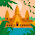but for 3rd question A shuld be the answer check itt..

1.Yes. You are right. It should be option A. Thanks for correcting this.

3.This website rocks
:) :))))))))

4.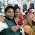Thanz fr uploading tis page,I read my portions in tis pge fr exam.
It helpd me usefully.

5.please write little big answer this is not enough for a 8grade student

6.for Q 13 you have given the ans of Q 14

7.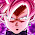Whers q 14 ans?

8.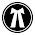answer for question 13 is missing. you have given the answer for question 14 to quetion 13

9.the formula of sphere oume is wrong in ques. 15.it should be:-
4/3 pi r cube

10.11.http://www.meritnation.com/cbse/class8/ncert-solutions/science/science---ncert-solution/stars-and-the-solar-system/page237-exercise-qno13/1295_96_8804/do-all-thestars-in-the-sky-move-explain

12.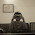for the question 13 u given the answer for question 14

13.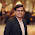improve your webpage and it also help me a lot

14.Very nice help

15.Very nice help

16.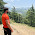Thanx for ur hlp

17.for Q13 you have give ans of Q14 .....check it

18.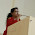all are wrong answers....

1.no read it again

19.where's question 11,12, and 14

20.amazing site

21.amazing site

22.Thank u for uploading thelse questions and thanxx for my help in my exams and it's a very fab site

23.Thank u for uploading thelse questions and thanxx for my help in my exams and it's a very fab site

24.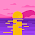good
fabulous

25.26.